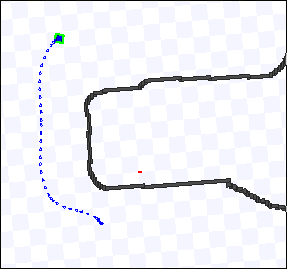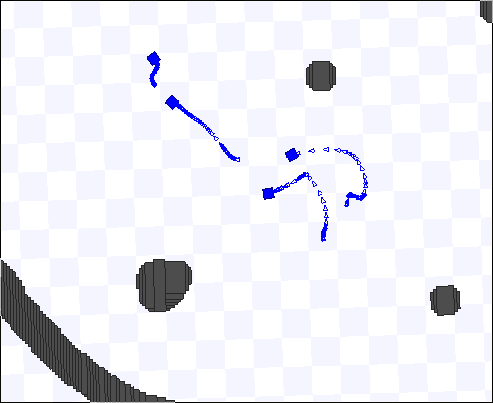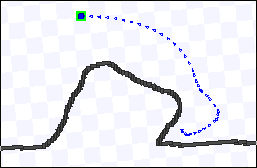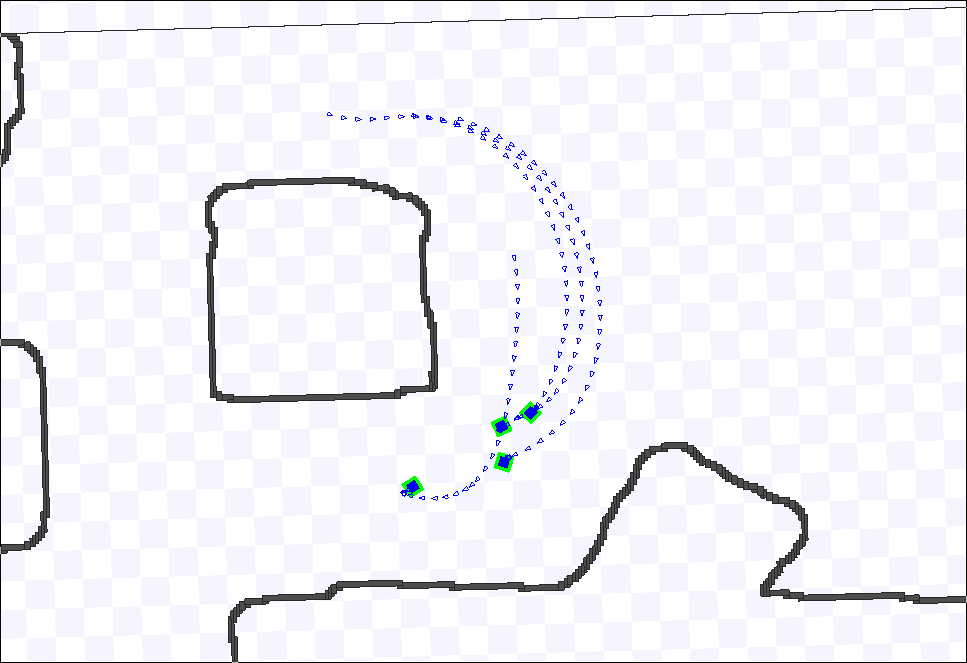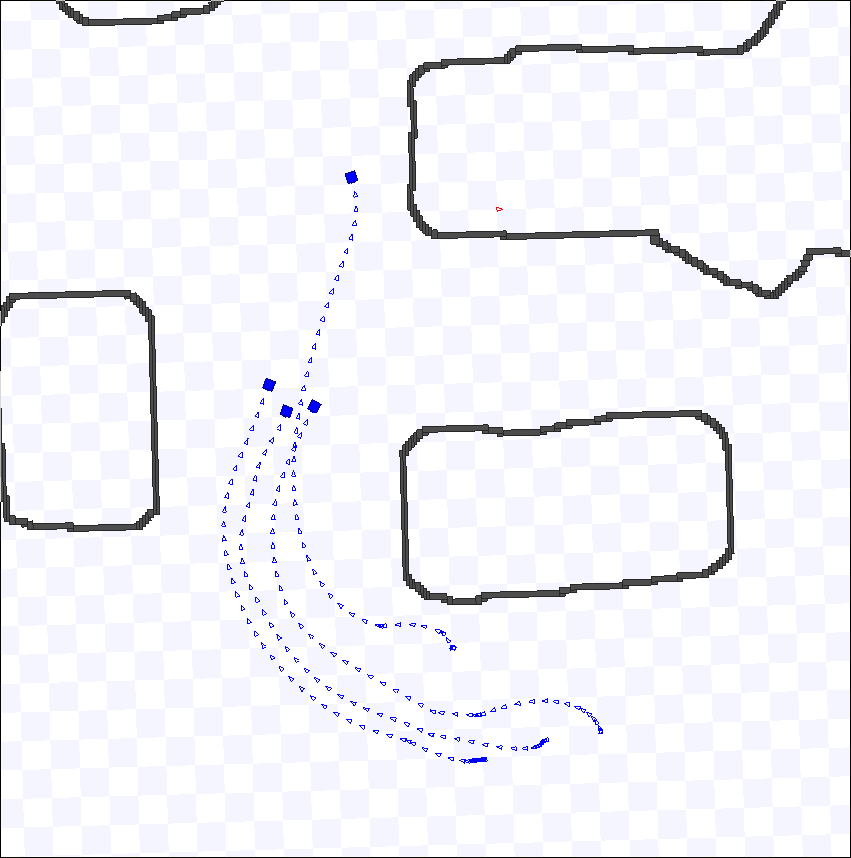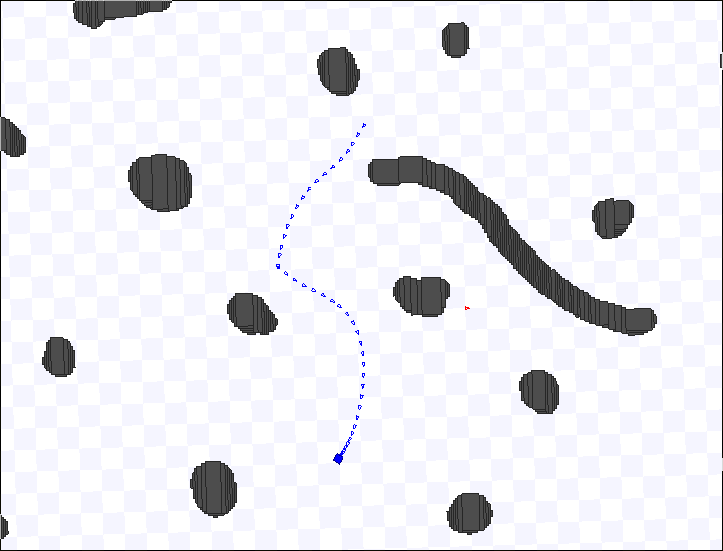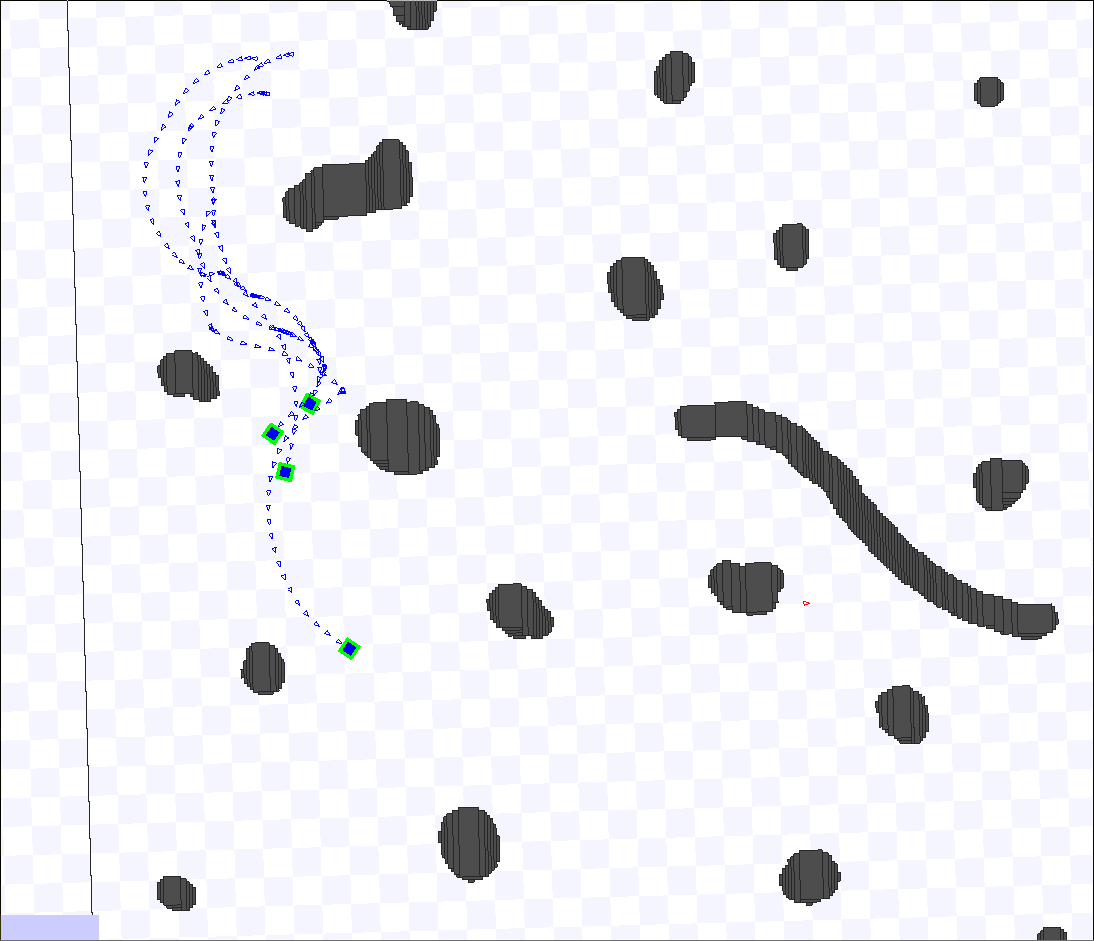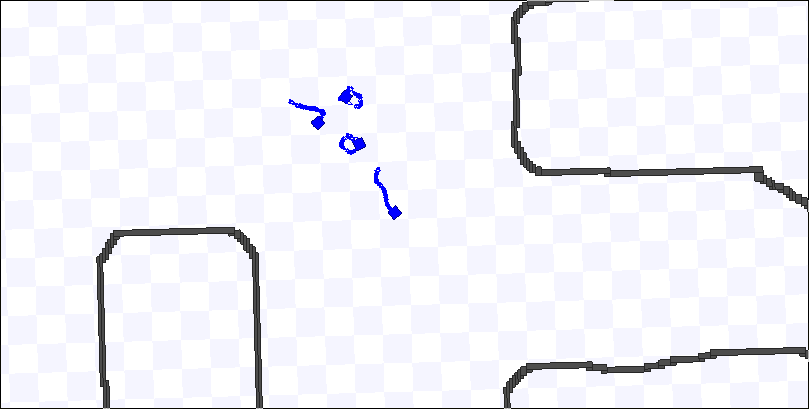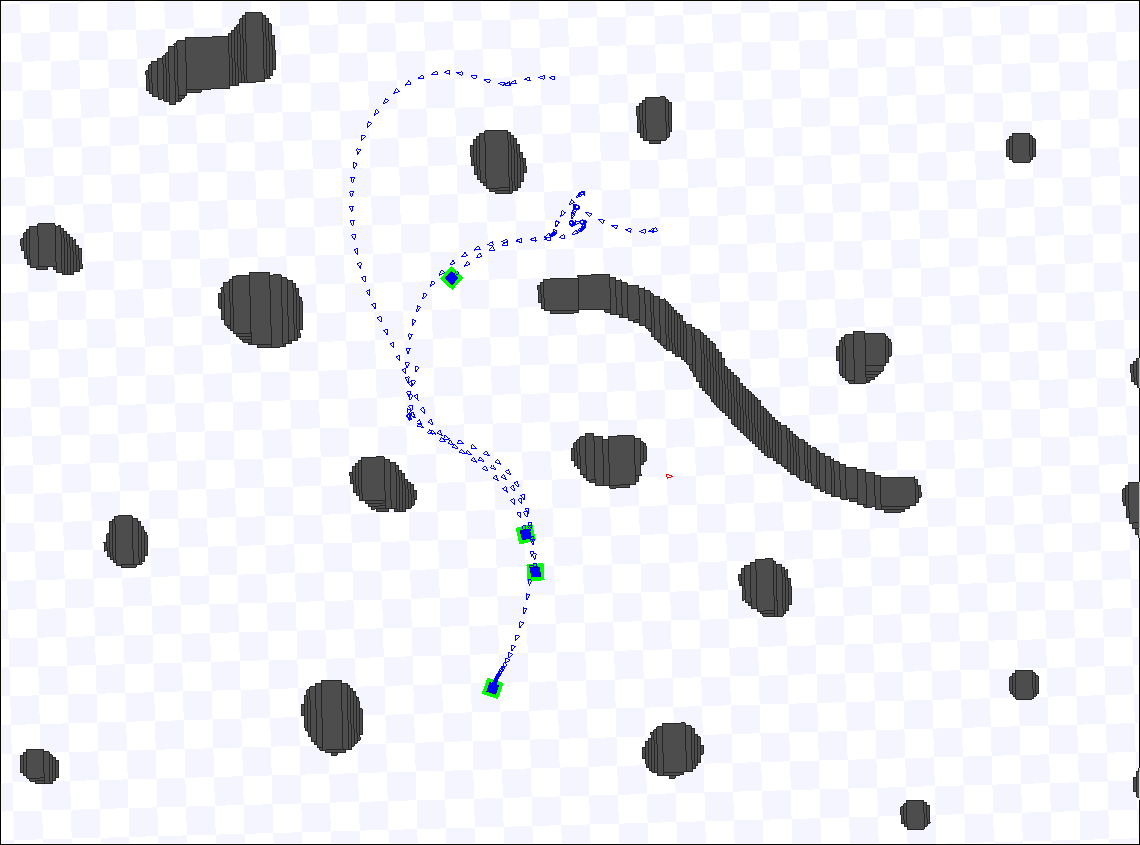# Potential Fields

#### 1. Provided Tunable Parameters

The following parameters were provided in the given code:

{Parameters 1}
// Tunable motion controller parameters
const static double FORWARD_SPEED_MPS = 2.0;
const static double ROTATE_SPEED_RADPS = M_PI;


See also sections 4, 5, 7, 8, 11, 13, 15 and 16

This code is used in section 18

FORWARD_SPEED_MPS defines the forward speed of the robot in meters per second. ROTATE_SPEED_RADPS defines the rotation speed of the robot in radians per second.

#### 2. Physical Vectors

{Structs 2}
{Vector Struct, 2}


This code is used in section 18

The following struct provides a data structure for vectors in two-dimensional space. Simple assignment and operator-assignment methods for 2D vectors will be useful later for calculating total force of the potential fields and generating random forces, and so are also included. They are so close to boilerplate that they are unlabelled.

{Vector Struct 2}
struct Vector2 {
double x;
double y;

};


This code is used in section 2

Vector2 &operator=(const Vector2 &v) {x=v.x; y=v.y; return *this;}
Vector2 &operator+=(const Vector2 &v) {x+=v.x; y+=v.y; return *this;}
const Vector2 operator+(const Vector2 &v) {return Vector2(*this)+=v;}
Vector2 &operator-=(const Vector2 &v) {x-=v.x; y-=v.y; return *this;}
const Vector2 operator-(const Vector2 &v) {return Vector2(*this)-=v;}
Vector2 &operator=(const double &a) {x=y=a; return *this;}
Vector2 &operator*=(const double &a) {x*=a; y*=a; return *this;}
const Vector2 operator*(const double &a) {return Vector2(*this)*=a;}
bool operator==(const Vector2 &v) {return x==v.x && y==v.y;}
bool operator!=(const Vector2 &v) {return !(*this == v);}


This code is used in section 2

#### 3. Potential Fields Introduction

Potential fields are composed of attractive and repulsive forces. The formulas for the attractive forces are different for leaders and followers, whereas the formula for the repulsive forces is the same for all robots.

{Methods 4}
{Leader Attraction Method, 4}


See also sections 5, 6, 7, 8, 10, 11, 12, 13, 14, 15, 16 and 17

This code is used in section 18

For the leader, the attractive force is

\|F_a\| = \gamma \cdot \|S\|^2

\angle F_a = \angle S

S = X_\text{goal} - X_\text{robot}

where

• F_a is the attractive force vector.
• \gamma is the attraction constant.
• X_\text{goal} is the position vector of the goal.
• X_\text{robot} is the position vector of the robot.

This can be converted to rectangular coordinates.

\begin{array}{rcl} F_{a,x} & = & \|F_a\| \cdot \cos(\angle F_a) \\[0.5em] ~ & = & \gamma \cdot \|S\|^2 \cdot \cos(\angle S) \\[0.5em] ~ & = & \gamma \cdot \|S\| \cdot S_x \\[0.5em] ~ & = & k_a \cdot S_x \end{array}

\begin{array}{rcl} F_{a,y} & = & \|F_a\| \cdot \sin(\angle F_a) \\[0.5em] ~ & = & k_a \cdot S_y \end{array}

k_a = \gamma \cdot \|S\|

since

\|V\| = \sqrt{V_x^2 + V_y^2}
\angle V = \text{atan2}(V_y, V_x)

\cos(\angle V) = {V_x \over \|V\|}
\sin(\angle V) = {V_y \over \|V\|}

This combined calculation and conversion is far more efficient than doing them separately (the vector would have needed to be converted for addition anyway). This is easily implemented in C++.

/*
gamma:  attraction coefficient
gx, gy: position of the goal
rx, ry: position of the robot
*/
double gx, double gy, double rx, double ry) {
// Create the return struct
Vector2 fa;
// Get the distance to the goal
double sx = gx - rx;
double sy = gy - ry;
// Calculate the vector conversion factor
double ka = gamma * sqrt(sx*sx + sy*sy);
// Calculate the vector of the force
fa.x = ka * sx;
fa.y = ka * sy;
return fa;
}


This code is used in section 4

sqrt is from the cmath header (as are cos, sin, atan2, and M_PI), so that is included.

#include <cmath>


This code is used in section 18

The method is overloaded to use values from the object.

Vector2 getLeaderAttraction() {
Pose &robot = pose[ID];
goalX, goalY, robot.x, robot.y);
}


This code is used in section 4

The attraction constant \gamma is a const parameter.

{Parameters 1} +=
// Parameters added for leader attraction
const static double ATTRACTION_CONSTANT_GAMMA = 1.0;


This code is used in section 18

With compiler optimization, this value will never need to be copied as an argument, since it will be inlined into the function (further discussion can be found here).

#### 5. Follower Attractive Force

{Methods 4} +=
{Follower Attraction Method, 5}


See also sections 6, 7, 8, 10, 11, 12, 13, 14, 15, 16 and 17

This code is used in section 18

For followers, the attractive force is

\|F_a^j\|=\begin{cases} -{\alpha \over d_{j \to 0}^2}, & \text{if }d_{j \to 0} < d_\text{safe} \\ 0, & \text{if }d_\text{safe} < d_{j \to 0} < d_\text{far} \\ \gamma \cdot d_{j \to 0}^2, & \text{otherwise} \end{cases}

\angle F_a^j = \angle S_{j \to 0}

d_{j \to 0} = ||S_{j \to 0}|| - (r_0 + r_j)

S_{j \to 0} = X_0 - X_j

where

• F_a^j is the attractive force vector for robot j.
• \alpha is the repulsion constant.
• X_n is the position vector of robot n.
• r_n is the radius of robot n.
• d_\text{safe} is the closest safe distance between a robot and obstacle.
• d_\text{far} is the distance that is "too far" from the leader.
• \gamma is the attraction constant.

Note that {\alpha \over d_{j \to 0}^2} has been made negative. This is because it does not make sense to be attracted to the leader when you are dangerously close to the leader. This does not actually affect the magnitude, instead reversing the angle, but the negation on the magnitude formula is both notationally simpler and closer to the actual implementation.

This can be converted to rectangular coordinates.

\begin{array}{rcl} F_{a,x}^j & = & \|F_a^j\| \cdot \cos(\angle F_a^j) \\[0.5em] ~ & = & k_a^j \cdot S_{j \to 0}^x \end{array}

\begin{array}{rcl} F_{a,y}^j & = & \|F_a^j\| \cdot \sin(\angle F_a^j) \\[0.5em] ~ & = & k_a^j \cdot S_{j \to 0}^y \end{array}

k_a^j = \begin{cases} -{\alpha \over d_{j \to 0}^2 \cdot \|S_{j \to 0}\|}, & \text{if }d_{j \to 0} < d_\text{safe} \\ 0, & \text{if }d_\text{safe} < d_{j \to 0} < d_\text{far} \\ {\gamma \cdot d_{j \to 0}^2 \over \|S_{j \to 0}\|}, & \text{otherwise} \end{cases}

In C++:

{Follower Attraction Method 5}
/*
alpha:    repulsion coefficient
gamma:    attraction coefficient
x0x, x0y: position of robot 0
xjx, xjy: position of robot j
dSafe:    safe distance
dFar:     "too far" distance
*/
Vector2 getFollowerAttraction(double alpha, double gamma,
double x0x, double x0y, double xjx, double xjy, double r0, double rj,
double dSafe, double dFar) {
// Create the return struct
Vector2 fa;
// Calculate the distance between the center of robot j to 0
double sx = x0x - xjx;
double sy = x0y - xjy;
double sm = sqrt(sx*sx + sy*sy);
// Subtract the radii of the robots
double d = sm - (r0 + rj);
// Calculate the vector conversion factor
double ka;
if (d < dSafe) {
ka = -alpha / (d*d * sm);
} else if (d < dFar) {
fa.x = 0;
fa.y = 0;
return fa;
} else {
ka = gamma * d*d / sm;
}
// Calculate the vector of the force
fa.x = ka * sx;
fa.y = ka * sy;
return fa;
}


This code is used in section 5

Overloaded to use values from the object:

{Follower Attraction Method 5} +=
Vector2 getFollowerAttraction() {
Pose &x0 = pose;
Pose &xj = pose[ID];
return getFollowerAttraction(REPULSION_CONSTANT_ALPHA,
ATTRACTION_CONSTANT_GAMMA, x0.x, x0.y, xj.x, xj.y, ROBOT_RADIUS_M,
}


This code is used in section 5

The repulsion constant \alpha, the attraction constant \gamma, the robot radius, and the safe and far distances are all const parameters. \gamma has already been defined in "Leader Attractive Force" above.

{Parameters 1} +=
// Parameters added for follower attraction
const static double REPULSION_CONSTANT_ALPHA = 1.0;
const static double ROBOT_RADIUS_M = 0.3;
const static double SAFE_DISTANCE_M = 0.75;
const static double FAR_DISTANCE_M = 4.0;


This code is used in section 18

#### 6. General Attractive Force

{Methods 4} +=
{Attraction Method, 6}


See also sections 5, 7, 8, 10, 11, 12, 13, 14, 15, 16 and 17

This code is used in section 18

Now that there are algorithms for both the leader and follower attractive forces, a single method that will select which algorithm to use and return the result can be created.

{Attraction Method 6}
Vector2 getAttraction() {
if (ID == 0) {
} else {
return getFollowerAttraction();
}
}


This code is used in section 6

#### 7. Laser Repulsive Force

{Methods 4} +=
{Repulsion Method, 7}


See also sections 5, 6, 8, 10, 11, 12, 13, 14, 15, 16 and 17

This code is used in section 18

The repulsive force is

\|F_r^i\|=\begin{cases} {\alpha \over (d_i - d_\text{safe})^2}, & \text{if }d_\text{safe} + \epsilon < d_i < \beta \\[1em] {\alpha \over \epsilon^2}, & \text{if }d_i < d_\text{safe} + \epsilon \\ 0, & \text{otherwise} \end{cases}

\angle F_r^i = \angle S_i

d_i = \|S_i\|

where

• F_r^i is the repulsive force for laser range reading i.
• \alpha is the repulsion constant.
• S_i is the vector from the remote end of the laser reading to the sensor.
• d_\text{safe} is the closest safe distance between a robot and obstacle.
• \epsilon is the error constant.
• \beta is the relevance distance.

Rectangular coordinates:

\begin{array}{rcl} F_{r,x}^i & = & \|F_r^i\| \cdot \cos(\angle F_r^i) \\[0.5em] ~ & = & \|F_r^i\| \cdot \cos(\angle S_i) \end{array}

\begin{array}{rcl} F_{r,y}^i & = & \|F_r^i\| \cdot \sin(\angle F_r^i) \\[0.5em] ~ & = & \|F_r^i\| \cdot \sin(\angle S_i) \end{array}

This conversion may seem less simplified than those for the attractive forces, but this is because S_i is obtained in polar coordinates, so this formulation is simpler. This is easily implemented in C++.

{Repulsion Method 7}
/*
alpha:    repulsion coefficient
sim, siTheta: vector from end of laser (on wall) to sensor
dSafe:    safe distance
epsilon:  error constant
beta:     relevance distance
*/
Vector2 getRepulsion(double alpha, double sim, double siTheta, double dSafe,
double epsilon, double beta) {
// Create the return struct
Vector2 fr;
// Calculate the magnitude of the force
double frm;
if (sim < dSafe + epsilon) {
frm = alpha / (epsilon*epsilon);
} else if (sim < beta) {
double di_ds = sim - dSafe;
frm = alpha / (di_ds*di_ds);
} else {
fr.x = 0;
fr.y = 0;
return fr;
}
fr.x = frm * cos(siTheta);
fr.y = frm * sin(siTheta);
return fr;
}


This code is used in section 7

Overloaded to use values from the object:

{Repulsion Method 7} +=
/*
i:  index of the laser range reading
*/
Vector2 getRepulsion(size_t i) {
// Get the magnitude
float m = (*laserRanges)[i];
// Calculate the angle
double theta = laserAngleMin + i*laserAngleIncrement;
// Calculate the vector
return getRepulsion(REPULSION_CONSTANT_ALPHA, m, theta,
SAFE_DISTANCE_M, ERROR_CONSTANT_EPSILON_M, RELEVANCE_DISTANCE_BETA_M);
}


This code is used in section 7

Obtaining the list of vector readings will be discussed in the next section, but for now, the member variables should be added.

{Laser Variables 7}
const std::vector<float> *laserRanges;
double laserAngleMin;
double laserAngleIncrement;


This code is used in section 9

Error constant \epsilon and relevance distance \beta need to be added to the const parameter list.

{Parameters 1} +=
// Parameters added for repulsion
const static double ERROR_CONSTANT_EPSILON_M = 0.03125;
const static double RELEVANCE_DISTANCE_BETA_M = 8.0;


This code is used in section 18

A method can be created for determining the total force, but to do so, the laser data needs to be parsed, and for the followers, the location of the leader on the scans needs to be determined so that the repulsive force will not apply to the leader. Thus the laser scan data must be handled before a total repulsion method can be designed.

#### 8. Other Repulsion

{Methods 4} +=
{Other Repulsion Patch Method, 8}


See also sections 5, 6, 7, 10, 11, 12, 13, 14, 15, 16 and 17

This code is used in section 18

Fix

Unfortunately, it seems that the other robots are not picked up by the laser as intended. To solve this problem, an extra repulsive force will be added for the other (non-leader) robots. The existing repulsion algorithm can be used for this. All that is required is that a polar vector be constructed from the other robot to this node's robot. If this robot is m and the other is n, then this is

S_{n \to m} = X_m - X_n

In C++:

{Other Repulsion Patch Method 8}
Vector2 getOtherRepulsion() {
// Create the return vector
Vector2 fp;
// Add all the repulsive forces
Pose &m = pose[ID];
for (int i = 1; i < numRobots; ++i) {
if (i == ID) continue;
Pose &n = pose[i];
double sx = m.x - n.x;
double sy = m.y - n.y;
fp += getRepulsion(REPULSION_CONSTANT_ALPHA, sqrt(sx*sx + sy*sy),
atan2(sy, sx), SAFE_DISTANCE_M, ERROR_CONSTANT_EPSILON_M,
RELEVANCE_DISTANCE_BETA_M);
}
// Apply the factor
double factor = OTHER_REPULSION_FACTOR;
// Got a weird linker error if this wasn't put in a local variable
fp *= factor;
return fp;
}


This code is used in section 8

{Parameters 1} +=
// Other Repulsion
const static double OTHER_REPULSION_FACTOR = 2.0;


This code is used in section 18

#### 9. Parsing Laser Scans

{Member Variables 9}
{Laser Variables, 7}


This code is used in section 18

The first of the TODO-marked sections was the laser scan callback. The provided TODO comment was:

TODO: parse laser data (see http://www.ros.org/doc/api/sensor_msgs/html/msg/LaserScan.html)

There are two things to be done here: parsing the messages, and converting the relative angles into absolute angles — i.e. 0 along the positive x-axis, increasing counterclockwise, referring to the angle of the vector from the endpoint of the laser along the obstacle back to the sensor (the reverse of what the message angles refer to). This has a simple conversion formula.

\angle S_i = \theta + \phi + \pi

where

• S_i is the vector from the obstacle laser endpoint to the sensor.
• \theta is the heading of the robot.
• \phi is the relative (to the heading) angle of the laser reading.

This can be implemented as such:

{Laser Callback 9}
laserAngleMin = pose[ID].heading + msg->angle_min + M_PI;
laserAngleIncrement = msg->angle_increment;


This code is used in section 18

These two values can be used to determine the absolute angle for any range reading. To avoid unnecessary memory copying, only a pointer to the range vector will be copied.

{Laser Callback 9} +=
laserRanges = &msg->ranges;


This code is used in section 18

This does bring with it the risk that the vector will be deleted. Thankfully, the message is passed using a smart pointer, so keeping a reference will prevent it from being destroyed. Whenever the message is replaced, the old message will be deleted, and the new one will be preserved (that is, until the next message).

{Laser Variables 7} +=
sensor_msgs::LaserScan::ConstPtr laserMessage;


This code is used in section 9

{Laser Callback 9} +=
laserMessage = msg;


This code is used in section 18

{Structs 2} +=
{Leader Struct, 10}


This code is used in section 18

The followers need to remove the leader from their laser scan data. To do this, they need to determine where in the scan the leader is. Two methods can be used to do the filtering: one to be called once per spin, to do the pre-calculations, and another to be called for each point on the laser scan.

{Methods 4} +=
{Locate Leader Method, 10}


See also sections 5, 6, 7, 8, 11, 12, 13, 14, 15, 16 and 17

This code is used in section 18

As output from the location method and input to the checking method, there needs to be a struct for holding the values.

struct Leader {
double distanceThreshold;
double thetaMin;
double thetaMax;
};


This code is used in section 10

The checking method checks if the angle of the reading is within the section of the scan that contains the leader, and then compares the distances to check if the reading is actually an obstacle or not. If the reading is closer than the leader, then there is an obstacle between the leader and the follower, and the reading should not be ignored.

/*
laserAngle: absolute angle of the reading
*/
float laserRange, double laserAngle) {
}


This code is used in section 10

{Check for Leader Method 10} +=
bool checkForLeader(const Leader &leader, size_t i) {
// Get the magnitude
float m = (*laserRanges)[i];
// Calculate the angle
double theta = laserAngleMin + i*laserAngleIncrement;
}


This code is used in section 10

To locate the leader, the formulas are:

d_\text{threshold} = \|S_{j \to 0}\| - (r_0 + \epsilon)

\theta_\text{min} = \angle S_{j \to 0} - \Delta \theta

\theta_\text{max} = \angle S_{j \to 0} + \Delta \theta

\Delta \theta = |~\text{atan2}(r_0, \|S_{j \to 0}\|)~|

S_{j \to 0} = X_0 - X_j

where

• d_\text{threshold} is the threshold laser range for the leader.
• \theta_\text{min} is the minimum angle to be considered part of the leader.
• \theta_\text{max} is the maximum angle to be considered part of the leader.
• X_n is the position vector of robot n.
• \epsilon is the error constant.
/*
x0x, x0y: position of robot 0
xjx, xjy: position of robot j
epsilon:  error constant
*/
double xjx, double xjy, double r0, double epsilon) {
// Calculate the distance between the center of robot j to 0
double sx = x0x - xjx;
double sy = x0y - xjy;
double sm = sqrt(sx*sx + sy*sy);
double sTheta = atan2(sy, sx);
// Calculate the threshold distance
leader.distanceThreshold = sm - (r0 + epsilon);
// Calculate the angle difference
double dTheta = std::abs(atan2(r0, sm));
// Calculate the min and max angles
}


This code is used in section 10

Overloaded to use values from the object:

void locateLeader(Leader &leader) {
Pose &x0 = pose;
Pose &xj = pose[ID];
ERROR_CONSTANT_EPSILON_M);
}


This code is used in section 10

#### 11. Random Force

{Methods 4} +=
{Position EMA Reset Method, 11}
{Position EMA Update Method, 11}
{Random Force Generator, 11}
{Random Angle Generator, 11}


See also sections 5, 6, 7, 8, 10, 12, 13, 14, 15, 16 and 17

This code is used in section 18

A random force is added to the total to avoid getting stuck in local minima. The magnitude of the force should increase as the robot stays put more. "Staying put more" is terribly vague, so a "staying put index" will be defined as the inverse of the distance between the robot's position and an exponential moving average (EMA) of the robot's position.

p_t = {1 \over \|Y_t - E_t\|}

E_0 = Y_0

E_t = \eta \cdot Y_t + (1-\eta) \cdot E_{t-1}

where

• p_t is the "staying put index" at timestep t.
• Y_t is the position vector of the robot at time t.
• E_t is the EMA of the robot's position at time t.
• \eta is the EMA coefficient.

To calculate the EMA at every time step, the previous EMA must be stored.

{Member Variables 9} +=
Vector2 positionEMA;


This code is used in section 18

Whenever a new goal is set (and at the beginning of the program), the EMA needs to be reset to the current position.

{Position EMA Reset Method 11}
void resetPositionEMA() {
// Reset to the robot's current position
Pose &robot = pose[ID];
positionEMA.x = robot.x;
positionEMA.y = robot.y;
}


This code is used in section 11

At the beginning of the program, the pose data for the robot has to be retrieved before it can be used to reset the EMA.

{Post-Initialization 11}
// Get pose data
ros::Time epoch(0.0);
while (pose[ID].t == epoch && ros::ok())
ros::spinOnce();
// Reset EMA to pose
resetPositionEMA();


This code is used in section 18

To update the EMA, the formula described above is implemented.

{Position EMA Update Method 11}
void updatePositionEMA() {
// Discount the old value
positionEMA *= 1 - EMA_COEFFICIENT_ETA;
Pose &robot = pose[ID];
positionEMA.x += EMA_COEFFICIENT_ETA * robot.x;
positionEMA.y += EMA_COEFFICIENT_ETA * robot.y;
}


This code is used in section 11

The random force is generated from the "staying put index" (described above) by the following formulas.

\|F_\text{random}\| = \zeta \cdot p_t

\angle F_\text{random} = u_{[-\pi,\pi)}

where

• F_\text{random} is the random force.
• \zeta is the conversion constant.
• p_t is the current "staying put index."
• u_{[-\pi,\pi)} is on a uniform distribution on the interval [-\pi,\pi).

In rectangular coordinates:

F_\text{random}^x = \zeta \cdot p_t \cdot \cos(u_{[-\pi,\pi)})

F_\text{random}^y = \zeta \cdot p_t \cdot \sin(u_{[-\pi,\pi)})

In C++:

{Random Force Generator 11}
/*
zeta: conversion constant
p:    "staying put index"
*/
Vector2 generateRandomForce(double zeta, double p) {
// Create the return struct
Vector2 f;
// Generate the random angle
double u = generateRandomAngle();
// Calculate the force
double k = zeta * p;
f.x = k * cos(u);
f.y = k * sin(u);
return f;
}


This code is used in section 11

Overloaded to use values from the object:

{Random Force Generator 11} +=
Vector2 generateRandomForce() {
// Calculate the "staying put index"
Pose &robot = pose[ID];
double ipx = robot.x - positionEMA.x;
double ipy = robot.y - positionEMA.y;
double p = 1 / sqrt(ipx*ipx + ipy*ipy);
// Generate the force
return generateRandomForce(CONVERSION_CONSTANT_ZETA, p);
}


This code is used in section 11

The EMA coefficient \eta and the conversion constant \zeta are const parameters.

{Parameters 1} +=
// Parameters added for random force
const static double EMA_COEFFICIENT_ETA = 0.0625;
const static double CONVERSION_CONSTANT_ZETA = 0.125;


This code is used in section 18

u_{[-\pi,\pi)} is generated using rand. It has some nonuniformity issues on some systems, and any simple conversion into a floating-point uniform distribution (as below) introduce nonuniformities on all systems, but ROS nodes are typically compiled with C++03, which does not have the STL header random, which provides far superior RNG tools.

{Random Angle Generator 11}
double generateRandomAngle() {
return (double(rand()) / RAND_MAX)*2*M_PI - M_PI;
}


This code is used in section 11

The generator is seeded in post-initialization.

{Post-Initialization 11} +=
// Initialize random time generator
srand(time(NULL));


This code is used in section 18

A personal note: rand has all kinds of problems, and I really dislike using it in this way, but I'm not going to port everything to C++11 so that I can get a properly uniform distribution. I just wanted to note that this is NOT a good way to generate a reliably uniform distribution of floating-point numbers.

#### 12. Total Force

{Methods 4} +=
{Total Force Method, 12}


See also sections 5, 6, 7, 8, 10, 11, 13, 14, 15, 16 and 17

This code is used in section 18

The formula for total force is fairly simple.

F_T = \sum_i F_r^i + F_a + F_\text{random}

It is slightly complicated by filtering the laser data (as described in the previous section), but it is still fairly straightforward to implement in C++.

{Total Force Method 12}
Vector2 getTotalForce() {
// Create the return struct, initialized with the attractive force.
Vector2 ft = getAttraction();
ft += generateRandomForce();
// Get the number of laser data points
size_t len = laserRanges->size();
if (ID == 0) {
// Leader does not need to filter for itself.
for (size_t i = 0; i < len; ++i) {
ft += getRepulsion(i);
}
} else {
// Followers need to filter out the leader.
for (size_t i = 0; i < len; ++i) {
ft += getRepulsion(i);
}
}
}
// Patch because laser doesn't hit other robots
ft += getOtherRepulsion();
// Return the total force
return ft;
}


This code is used in section 12

#### 13. Force to Velocity

{Methods 4} +=
{Angular Velocity Method, 13}
{Linear Velocity Method, 13}
{Sign Function, 13}


See also sections 5, 6, 7, 8, 10, 11, 12, 14, 15, 16 and 17

This code is used in section 18

The velocity for the robot is

\omega = \text{sgn}(\omega_\text{raw}) \cdot \min(|\omega_\text{raw}|, \omega_\text{max})

\omega_\text{raw} = \kappa \cdot \theta_d

v = \begin{cases} \min(v_\text{raw}, v_\text{max}), & \text{if }v_\text{raw} > 0 \\ 0, & \text{otherwise} \end{cases}

v_\text{raw} = \lambda \cdot \|F_T\| \cdot \cos \theta_d

\theta_d = \text{reduceAngle}(\angle F_T - \theta_r)

\text{reduceAngle}(\phi) = \text{atan2}(\sin(\phi), \cos(\phi))

\text{sgn}(a) = {a \over |a|}

where

• \omega is the capped angular velocity.
• \omega_\text{raw} is the uncapped angular velocity.
• \omega_\text{max} is the maximum allowable angular speed.
• \kappa is the angular scaling constant.
• v is the capped linear velocity.
• v_\text{raw} is the uncapped linear velocity.
• v_\text{max} is the maximum allowable linear speed.
• \lambda is the linear scaling constant.
• F_T is the total force.
• \theta_r is the heading of the robot.

The following definition for \text{reduceAngle} was also tried, but the implementation did not seem to work in that it would sometimes return values with absolute values greater than \pi.

\text{reduceAngle}(\phi) = \text{fmod}(\phi + \pi, 2\pi) - \pi

\text{fmod}(n, d) = n - \lfloor ^n/_d \rfloor \cdot d

In C++:

{Angular Velocity Method 13}
/*
kappa:    angular scaler
theta:    angle between force and heading
omegaMax: max allowable angular speed
*/
double getAngularVelocity(double kappa, double theta, double omegaMax) {
double raw = kappa * theta;
return sgn(raw) * std::min(std::abs(raw), omegaMax);
}


This code is used in section 13

{Linear Velocity Method 13}
/*
lambda:   linear scaler
ftx, fty: total force
theta:    angle between force and heading
vMax:     max allowable linear speed
*/
double getLinearVelocity(double lambda, double ftx, double fty,
double theta, double vMax) {
double raw = lambda * sqrt(ftx*ftx + fty*fty) * cos(theta);
return (raw > 0) ? std::min(raw, vMax) : 0;
}


This code is used in section 13

{Sign Function 13}
double sgn(double a) {
return (a > 0) - (a < 0);
}


This code is used in section 13

Overloaded to use values from the object (except in calculating \theta_d and F_T, as those are to be calculated further up the callstack for efficiency):

{Angular Velocity Method 13} +=
double getAngularVelocity(double theta) {
}


This code is used in section 13

{Linear Velocity Method 13} +=
double getLinearVelocity(Vector2 &ft, double theta) {
return getLinearVelocity(LINEAR_SCALER_LAMBDA, ft.x, ft.y, theta,
FORWARD_SPEED_MPS);
}


This code is used in section 13

std::min is in the header algorithm.

#include <algorithm>  // For min


This code is used in section 18

The scaling constants \kappa and \lambda are const parameters:

{Parameters 1} +=
// Parameters added for force to velocity
const static double ANGULAR_SCALER_KAPPA = 0.25;
const static double LINEAR_SCALER_LAMBDA = 0.0625;


This code is used in section 18

The second of the TODO-marked sections was the laser scan callback. The provided TODO comment was:

TODO: remove demo code, compute potential function, actuate robot

Demo code: print each robot's pose

 for (int i = 0; i < numRobots; i++) {
ROS_DEBUG_STREAM(i << " " << "Pose: " << pose[i].x << ", " << pose[i].y
<< ", " << pose[i].heading << std::endl);
}


Most of the necessary components for doing this have already been defined above, so implementing this final step is fairly straightforward.

{Actuate Robot 13}
// Update the EMA for the random force
updatePositionEMA();
// Calculate the total force
Vector2 ft = getTotalForce();
// Calculate the shortest angle between the force and the heading
double theta = atan2(ft.y, ft.x) - pose[ID].heading;
theta = atan2(sin(theta), cos(theta));
// Calculate the velocities
double omega = getAngularVelocity(theta);
double v = getLinearVelocity(ft, theta);
// Move the robot
move(v, omega);


This code is used in section 18

#### 14. Timing

The following code provides a method for executing code less than every tick.

{Member Variables 9} +=
unsigned tickCounter;


This code is used in section 18

{Post-Initialization 11} +=
tickCounter = 0;


This code is used in section 18

{Actuate Robot 13} +=
++tickCounter;


This code is used in section 18

{Methods 4} +=
bool checkFrequency(float hz) {
return tickCounter % int(30.0 / hz) == 0;
}


See also sections 5, 6, 7, 8, 10, 11, 12, 13, 15, 16 and 17

This code is used in section 18

#### 15. Detecting the Goal

{Methods 4} +=
{Is on Goal Method, 15}


See also sections 5, 6, 7, 8, 10, 11, 12, 13, 14, 16 and 17

This code is used in section 18

Due to the random force, interactions with other robots, and rounding errors, the leader will not ever be right on the goal, so to detect goal achievement, it must be defined as an area. A robot has reached the goal if

\|S_\text{goal}\| < \sigma

S_\text{goal} = X_\text{goal} - E_t

where

• X_\text{goal} is the position vector of the goal.
• E_t is the EMA vector of the robot's position.
• \sigma is the radius of the goal.

In C++:

{Is on Goal Method 15}
bool isOnGoal(double gx, double gy, double ex, double ey, double sigma) {
double sx = gx - ex;
double sy = gy - ey;
return sqrt(sx*sx + sy*sy) < sigma;
}


This code is used in section 15

Overloaded to use values from the object:

{Is on Goal Method 15} +=
bool isOnGoal() {
return isOnGoal(goalX, goalY, positionEMA.x, positionEMA.y,
}


This code is used in section 15

The goal radius \sigma is a const parameter.

{Parameters 1} +=
const static double GOAL_RADIUS_SIGMA_M = 1.25;


This code is used in section 18

This code was used for debugging purposes, and is not used for the main functionality of the program.

#### 16. Selecting a New Goal

{Methods 4} +=
{Goal Generator, 16}


See also sections 5, 6, 7, 8, 10, 11, 12, 13, 14, 15 and 17

This code is used in section 18

A new goal is selected using the following formula.

X_\text{goal} = X_\text{robot} + R

\|R\| = u_{[0.5,1.5)}, \angle R = u_{[-\pi,\pi)}

where

• X_\text{goal} is the position vector of the goal.
• X_\text{robot} is the position vector of the robot.
• u_{[a,b)} is on a uniform distribution on the interval [a,b).

In C++:

{Goal Generator 16}
void generateGoal() {
// Generate random numbers
double um = 0.5 + (double(rand()) / RAND_MAX);
double ua = generateRandomAngle();
// Calculate new goal
Pose &robot = pose[ID];
goalX = robot.x + um * cos(ua);
goalY = robot.y + um * sin(ua);
// Reset EMA to pose
resetPositionEMA();
}


This code is used in section 16

This is called at startup and every 5 seconds.

{Post-Initialization 11} +=
if (ON_RANDOM_WALK && ID == 0) generateGoal();


This code is used in section 18

{Actuate Robot 13} +=
if (ON_RANDOM_WALK && ID == 0
&& checkFrequency(GOAL_REGENERATION_FREQUENCY_HZ)) {
generateGoal();
}


This code is used in section 18

{Parameters 1} +=
const static bool ON_RANDOM_WALK = false;
const static double GOAL_REGENERATION_FREQUENCY_HZ = 0.2;


This code is used in section 18

#### 17. Debug Logging

{Post-Initialization 11} +=
ROS_INFO("Post-initialization completed.");


This code is used in section 18

{Methods 4} +=
void printDiagnostic(const Vector2 &ft, double theta, double v, double omega) {
ROS_INFO("%2d Pose:%6.2f,%6.2f,%+7.4f; F:%+10.4f,%+10.4f (%+7.4f);"
" pEMA:%6.2f,%6.2f; onGoal:%1d", ID, pose[ID].x, pose[ID].y,
pose[ID].heading, ft.x, ft.y, atan2(ft.y, ft.x), theta, v, omega, goalX,
goalY, positionEMA.x, positionEMA.y, isOnGoal());
}


See also sections 5, 6, 7, 8, 10, 11, 12, 13, 14, 15 and 16

This code is used in section 18

{Actuate Robot 13} +=
//if (checkFrequency(2.0)) printDiagnostic();


This code is used in section 18

This code was used for debugging purposes, and is not used for the main functionality of the program.

#### 18. Given Code

The following code was provided for the project. Note that btQuaternion and btMatrix3x3 have been changed to tf::Quaternion and tf::Matrix3x3, respectively. This is because libbullet has removed the function btMatrix3x3::getRPY. Migration from libbullet to tf is discussed at bullet_migration on the ROS Wiki. Also changed is that stage does not append a namespace for each robot for the single-robot maps, so some if-statements were added to handle this problem (in main and PotFieldBot::PotFieldBot). Writes to std::cout have been changed to use /rosout.

{src/potential_field.cpp 18}
#include "ros/ros.h"
#include "nav_msgs/Odometry.h"
#include "geometry_msgs/Twist.h"
#include "sensor_msgs/LaserScan.h"
#include <vector>
#include <cstdlib>  // Needed for rand()
#include <ctime>    // Needed to seed random number generator with a time value
#include "tf/LinearMath/Quaternion.h" // Needed to convert rotation ...
#include "tf/LinearMath/Matrix3x3.h"  // ... quaternion into Euler angles

#include <ros/console.h>  // For rosout

struct Pose {
double x; // in simulated Stage units
double y; // in simulated Stage units
ros::Time t;    // last received time

// Construct a default pose object with the time set to 1970-01-01
Pose() : x(0), y(0), heading(0), t(0.0) {};

// Process incoming pose message for current robot
void poseCallback(const nav_msgs::Odometry::ConstPtr& msg) {
double roll, pitch;
x = msg->pose.pose.position.x;
y = msg->pose.pose.position.y;
tf::Quaternion q = tf::Quaternion(msg->pose.pose.orientation.x,
msg->pose.pose.orientation.y, msg->pose.pose.orientation.z,
msg->pose.pose.orientation.w);
};
};

{Structs, 2}

class PotFieldBot {
public:
// Construst a new Potential Field controller object and hook up
// this ROS node to the simulated robot's pose, velocity control,
// and laser topics
PotFieldBot(ros::NodeHandle& nh, int robotID, int n,  double gx, double gy)
: ID(robotID), numRobots(n), goalX(gx), goalY(gy) {
// Advertise a new publisher for the current simulated robot's
// velocity command topic (the second argument indicates that
// if multiple command messages are in the queue to be sent,
// only the last command will be sent)

// Subscribe to the current simulated robot's laser scan topic and
// tell ROS to call this->laserCallback() whenever a new message
// is published on that topic
laserSub = nh.subscribe("base_scan", 1, &PotFieldBot::laserCallback, this);

// Subscribe to each robot' ground truth pose topic
// and tell ROS to call pose->poseCallback(...) whenever a new
// message is published on that topic
for (int i = 0; i < numRobots; i++) {
pose.push_back(Pose());
}
if (numRobots == 1) {
poseSubs.push_back(nh.subscribe("/base_pose_ground_truth",
1, &Pose::poseCallback, &pose));
} else {
for (int i = 0; i < numRobots; i++) {
poseSubs.push_back(nh.subscribe("/robot_" +
boost::lexical_cast<std::string>(i) + "/base_pose_ground_truth",
1, &Pose::poseCallback, &pose[i]));
}
}
};

// Send a velocity command
void move(double linearVelMPS, double angularVelRadPS) {
geometry_msgs::Twist msg;
// The default constructor will set all commands to 0
msg.linear.x = linearVelMPS;
commandPub.publish(msg);
};

// Process incoming laser scan message
void laserCallback(const sensor_msgs::LaserScan::ConstPtr& msg) {

{Laser Callback, 9}

};

// Main FSM loop for ensuring that ROS messages are
// processed in a timely manner, and also for sending
// velocity controls to the simulated robot based on the FSM state
void spin() {
ros::Rate rate(30); // Specify the FSM loop rate in Hz
ROS_INFO("Entering spin.");

while (ros::ok()) { // Keep spinning loop until user presses Ctrl+C

{Actuate Robot, 13}

ros::spinOnce();
// Need to call this function often to allow ROS to process
// incoming messages
rate.sleep();
// Sleep for the rest of the cycle, to enforce the FSM loop rate
}
};

void postInitialization() {

{Post-Initialization, 11}

}

{Methods, 4}

{Parameters, 1}

protected:
ros::Publisher commandPub;
// Publisher to the current robot's velocity command topic
ros::Subscriber laserSub;
// Subscriber to the current robot's laser scan topic
std::vector<ros::Subscriber> poseSubs;
// List of subscribers to all robots' pose topics
std::vector<Pose> pose; // List of pose objects for all robots
int ID;                 // 0-indexed robot ID
int numRobots;          // Number of robots, positive value
double goalX, goalY;    // Coordinates of goal

{Member Variables, 9}

};

int main(int argc, char **argv) {
std::cout << "Entered main." << std::endl;
int robotID = -1, numRobots = 0;
double goalX, goalY;
bool printUsage = false;

// Parse and validate input arguments
if (argc <= 4) {
printUsage = true;
} else {
try {
robotID = boost::lexical_cast<int>(argv);
numRobots = boost::lexical_cast<int>(argv);
goalX = boost::lexical_cast<double>(argv);
goalY = boost::lexical_cast<double>(argv);

if (robotID < 0) { printUsage = true; }
if (numRobots <= 0) { printUsage = true; }
} catch (std::exception err) {
printUsage = true;
}
}
if (printUsage) {
ROS_FATAL_STREAM("Usage: " << argv <<
" [ROBOT_NUM_ID] [NUM_ROBOTS] [GOAL_X] [GOAL_Y]");
return EXIT_FAILURE;
}

ros::init(argc, argv, "potfieldbot_" + std::string(argv));
// Initiate ROS node
ROS_INFO("ROS initialized.");
if (numRobots == 1) {
ros::NodeHandle n;
// Create handle
ROS_INFO("Node handle created: Single Mode");
PotFieldBot robbie(n, robotID, numRobots, goalX, goalY);
// Create new random walk object
ROS_INFO("Node Initialized.");
robbie.postInitialization();
robbie.spin();  // Execute FSM loop
} else {
ros::NodeHandle n("robot_" + std::string(argv));
// Create named handle "robot_#"
ROS_INFO("Node handle created: Group Mode");
PotFieldBot robbie(n, robotID, numRobots, goalX, goalY);
// Create new random walk object
ROS_INFO("Node initialized.");
robbie.postInitialization();
robbie.spin();  // Execute FSM loop
}

return EXIT_SUCCESS;
};


#### 19. Results

The robots showed good ability to reach the goal, even when it involved circumnavigating obstacles. They did not run into obstacles or each other, and although the followers followed the leader very loosely, none of them lost their way for very long.